# Online Electronics and Communication Engineering Test - Communication Systems Test 1Loading Test...

Instruction:

• This is a FREE online test. DO NOT pay money to anyone to attend this test.
• Total number of questions : 20.
• Time alloted : 30 minutes.
• Each question carry 1 mark, no negative marks.
• DO NOT refresh the page.
• All the best :-).

1.

Which of the following has the highest input impedance?

A.
 CEB.
 CBC.
 CCD.
 Push pullExplanation:

CC connection has very high input impedance and low output impedance.

2.

Consider the following statements

1. The amplitude of an FM wave is constant
2. FM is more immune to noise than AM
3. FM broadcasts operate in upper VHF and UHF frequency ranges
4. FM transmitting and receiving equipments are simpler as compared to AM transmitting and receiving equipments
Which of the above are correct?

A.
 1, 2, 3, 4B.
 1, 2, 3C.
 2, 3, 4D.
 1, 3, 4Explanation:

FM equipments are complex as compared to AM equipments.

3.

In a high speed modem the data rate handled is about

A.
 1000 bpsB.
 5000 bpsC.
 20000 bpsD.
 50000 bpsExplanation:

When data rate is in the range of 2400 to about 10800 bits per second, modem is designated as high speed.

4.

24 telephone channels, each band limited to 3.4 kHz are to be time division multiplexed using PCM. If sampling frequency is 10 kHz and number of quantization levels is 128, the required bandwidth of PCM is

A.
 1.68 MHzB.
 240 kHzC.
 81.6 kHzD.
 3.072 MHzExplanation:

Bandwidth ≈ 24 (ln2 128) 10 kHz = 1.68 MHz.

5.

If the average power of radar transmitters is 2 kW and the peak power of the transmitter is 1000 kW, what will be the duty cycle?

A.
 0.002B.
 0.02C.
 0.001D.
 0.01Explanation: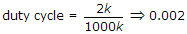.

6.

Some advantages of optical fibre cables are

1. small diameter
2. Immunity to cross talk and EM interference
3. Laser and LED modulation methods lend themselves ideally to digital operation
Which of the above are correct?

A.
 1 onlyB.
 1 and 2C.
 1, 2, 3D.
 2, 3Explanation:

Fibre optic cables have all these advantages.

7.

A signal m(t) with bandwidth 500 Hz is first multiplied by a signal g(t) where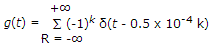. The resulting signal is then passes through an ideal low pass filter with bandwidth 1 kHz the output of the low pass filter would be

A.
 δ(t)B.
 m(t)C.
 0D.
 m(t) δ(t)Explanation:

Multiplication in time domain is equivalent to convolution. According to the property of impulse signal, convolution of a signal m(t) with δ(t) is signal itself.

m(t) x g(t)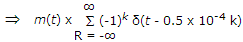m(t).

8.

Consider the following functions

1. It detects the AM video carrier IF so as to produce the video signal
2. It produces and separates out the inter carrier sound IF of 5.5 MHz
3. It amplifies the video signal
4. It amplifies the audio signal
The video detector in a colour TV performs which of the above functions

A.
 1, 2 and 3B.
 1, 2, 3 and 4C.
 1 and 2D.
 1, 2 and 4Explanation:

Video detector does not amplify any signal.

9.

In TV transmitter, the function of duplexer is

A.
 to amplify video signalB.
 to remove distortions in audio signalC.
 to combine outputs of visual and audio transmittersD.
 to amplify audio signalExplanation:

Duplexer combines video and audio signals so that they can be sent out by a common antenna.

10.

Microwave link repeaters are typically 50 km apart in TV transmission due to

A.
 atmosphere attenuationB.
 output power tube limitationsC.
 attenuation of surface waveD.
 earth's curvatureExplanation:

Earth's curvature limits line of sight distance. Therefore, repeaters are needed.

11.

The distance of a direct broadcasting satellite from earth's surface is about

A.
 1000 kmB.
 10000 kmC.
 36000 kmD.
 55000 km12.

For a three stage cascaded amplifier, having gains of G1, G2 and G3 and noise figures of F1, F2, F3 the overall noise figure

A.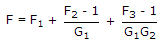B.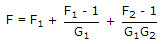C.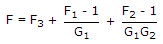D.
 None of the above13.

An SSB-AM signal cannot be demodulated by

A.
 Balanced modulatorB.
 Complete phase shift generatorC.
 Diode balanced modulatorD.
 Product detector14.

Ground wave can be used for communication upto

A.
 5 kHzB.
 12 kHzC.
 16 kHzD.
 25 kHz15.

In a hi-fi system the nonlinear distortion should be less than

A.
 10%B.
 5%C.
 2%D.
 1%16.

In a colour TV receiver SAW filter consists of

A.
 piezoelectric substrate on which are deposited two inter, digital transducersB.
 photo conductive substrate which are deposited two inter digital transducersC.
 photo voltaic substrate which are deposited two inter digital transducersD.
 photo resistive substrate which are deposited two inter digital transducers17.

A pulse width signal can be governed by

A.
 free running multi-vibratorB.
 monostable multi-vibratorC.
 Schmitt triggerD.
 JK flip-flop18.

The number of spectral component when two sine wave of same frequency multiplied is

A.
 2B.
 3C.
 ∞D.
 419.

In a square law modulator, the carrier frequency should be __________ times than the highest modulating frequency.

A.
 1B.
 2C.
 4D.
 320.

In a FM receiver, amplitude limiter

A.
 amplifiers low frequency signalsB.
 reduces the amplitude of signalsC.
 eliminates any change in amplitude of received FM signalsD.
 none of the above